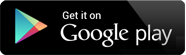## Video Lectures

Chapter 1 : Mathematical Modeling and Engineering Problem Solving
Topic : Mathematical Model, Conservation Laws and Engineering Problems
Content : Mathematical Modeling of Newton's 2nd Law of Motion 29 MB ,19:38 MIN , THEORY

Chapter 2 : Approximations and Round-off Errors
Topic : Significant Figures, Accuracy and Precision, Error Definitions, Round-off Error
Content : Absolute Errors, Relative Errors, Percentage Errors 45 MB ,32:36 MIN , THEORY

Chapter 3 : Truncation Errors and the Taylor Series
Topic : The Taylor Series, Error Propagation , Total Numerical Errors, Formulation Errors and Data Uncertainty
Content : Blunders 42 MB ,32:21 MIN , THEORY

Chapter 1 : Solution of Algebraic and Transcendental Equations
Topic : Introduction
Content : Algebraic and Transcendental Equations 7 MB ,4:54 MIN , THEORY

Chapter 1 : Solution of Algebraic and Transcendental Equations
Topic : The Bisection Method, The Regula-falsi Method
Content : Examples based on Bisection method, Examples based on Regula-falsi Method 26 MB ,18:41 MIN , THEORY

Chapter 1 : Solution of Algebraic and Transcendental Equations
Topic : The Newton-Raphson Method; The Secant Method
Content : Examples based on Newton-Raphson Method ; Examples based on Secant Method 24 MB ,17:31 MIN , THEORY

Chapter 2 : Interpolation
Topic : Forward Difference, Backward Difference
Content : Finite Difference; Forward Difference Operator; Backward difference operator ; Shift Operator; Relation between Forward , Backward and shift operators 38 MB ,28:18 MIN , THEORY

Chapter 2 : Interpolation
Topic : Newton's Forward Difference Interpolation, Newton's Backward Difference Interpolation, Lagrange's Interpolation
Content : Examples based on Newton's Forward and Newton's Backward Interpolation, Examples based on Lagrange's Interpolation 28 MB ,20:11 MIN , THEORY

Chapter 1 : Solutions of Simultaneous Algebraic Equations (Linear)
Topic : Gauss-Jordan Method, Gauss-Seidel Method
Content : Examples based on Gauss-Jordan and Gauss- Seidel method 42 MB ,29:5 MIN , THEORY

Chapter 2 : Numerical Differentiation and Integration
Topic : Numerical Differentiation
Content : Formula for Equally Spaced Values of the Arguments; Formula for Unqually Spaced Values of the Arguments; Examples based on Numerical Differentiation 21 MB ,15:25 MIN , THEORY

Chapter 2 : Numerical Differentiation and Integration
Topic : Numerical Integration Using Trapezoidal Rule, Simpson's 1/3rd and 3/8th Rule
Content : Examples based on Numerical Integration 15 MB ,11:13 MIN , THEORY

Chapter 1 : Numerical Solution of First and Second Order Differential Equations
Topic : Taylor Series , Euler's Method
Content : Examples based on Taylor Series Method and Euler's Method 35 MB ,25:51 MIN , THEORY

Chapter 1 : Numerical Solution of First and Second Order Differential Equations
Topic : Modified Euler's Method
Content : Examples based on Modified Euler's Method 15 MB ,11:6 MIN , THEORY

Chapter 4 : Numerical Solution of First and Second Order Differential Equations
Topic : Runge-Kutta Method for 1st and 2nd Order Differential Equations
Content : Examples based on Runge- Kutta Method 13 MB ,9:42 MIN , THEORY

Chapter 2 : LINEAR REGRESSION
Topic : LEAST-SQUARE REGRESSION
Content : LINEAR REGRESSION 5 MB ,3:8 MIN , THEORY + PRACTICAL

Chapter 2 : MULTIPLE LINEAR REGRESSION
Topic : LEAST -SQUARE REGRESSION
Content : MULTIPLE LINEAR REGRESSION 10 MB ,6:14 MIN , THEORY + PRACTICAL

Chapter 2 : Method of Least Square
Topic : LEAST-SQUARE REGRESSION
Content : GENERAL LEAST SQUARES 13 MB ,8:13 MIN , THEORY + PRACTICAL

Chapter 2 : Non-Linear Regression
Topic : Least-Square Regression
Content : Non-Linear Regression 14 MB ,9:7 MIN , THEORY + PRACTICAL

Chapter 1 : Linear Programing
Topic : LINEAR OPTIMIZATION PROBLEM
Content : INTRODUCTION 7 MB ,4:29 MIN , THEORY + PRACTICAL

Chapter 1 : Linear Programing
Topic : LINEAR PROGRAMMING
Content : FORMULATION-I 14 MB ,8:41 MIN , THEORY + PRACTICAL

Chapter 1 : Linear Programing
Topic : LINEAR PROGRAMING
Content : FORMULATION-II 9 MB ,5:41 MIN , THEORY + PRACTICAL

Chapter 1 : Linear Programing
Topic : LINEAR PROGRAMMING
Content : GRAPHICAL SOLUTION-I 32 MB ,21:33 MIN , THEORY + PRACTICAL

Chapter 1 : Linear Programing
Topic : LINEAR PROGRAMMING
Content : GRAPHICAL SOLUTION-II 29 MB ,18:35 MIN , THEORY + PRACTICAL

Chapter 1 : Partial Diffrential Equation
Topic : Numerical solution of Partial Differential Equation
Content : Classification of Partial Differential Equations of the second order 27 MB ,17:22 MIN , THEORY + PRACTICAL

Chapter 2 : Partial Diffrential Equation
Topic : Numerical Solutions of Partial Differential Equations
Content : Difference equation corresponding to Laplace Equation 32 MB ,19:24 MIN , MATHS SOLUTION

## The Shikshak App

The Shikshak App is an honest attempt to provide quality education.